HTML5 表单属性

HTML5 新的表单属性

HTML5 的 <form> 和 <input>标签添加了几个新属性.

<form>新属性：

• autocomplete

• novalidate

<input>新属性：

• autocomplete

• autofocus

• form

• formaction

• formenctype

• formmethod

• formnovalidate

• formtarget

• height and width

• list

• min and max

• multiple

• pattern (regexp)

• placeholder

• required

• step

<form> / <input> autocomplete 属性

autocomplete 属性规定 form 或 input 域应该拥有自动完成功能。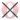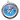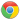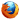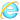实例

HTML form 中开启 autocomplete (一个 input 字段关闭 autocomplete ):

<form action="demo-form.php" autocomplete="on">
First name:<input type="text" name="fname"><br>
Last name: <input type="text" name="lname"><br>
E-mail: <input type="email" name="email" autocomplete="off"><br>
<input type="submit">
</form>

<form> novalidate 属性

novalidate 属性的一个boolean 属性.

novalidate 属性规定在提交表单时不应该验证 form 或 input 域。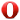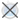实例

<form action="demo-form.php" novalidate>
E-mail: <input type="email" name="user_email">
<input type="submit">
</form>

<input> autofocus 属性

autofocus 属性是一个 boolean 属性.

autofocus 属性规定在页面加载时，域自动地获得焦点。实例

First name:<input type="text" name="fname" autofocus>

<input> form 属性

form 属性规定输入域所属的一个或多个表单。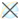实例

<form action="demo-form.php" id="form1">
First name: <input type="text" name="fname"><br>
<input type="submit" value="Submit">
</form>

Last name: <input type="text" name="lname" form="form1">

<input> formaction 属性

The formaction 属性用于描述表单提交的URL地址.

The formaction 属性会覆盖<form> 元素中的action属性.实例

<form action="demo-form.php">
First name: <input type="text" name="fname"><br>
Last name: <input type="text" name="lname"><br>
<input type="submit" value="Submit"><br>
</form>

<input> formenctype 属性

formenctype 属性描述了表单提交到服务器的数据编码 (只对form表单中 method="post" 表单)

formenctype 属性覆盖 form 元素的 enctype 属性。实例

<form action="demo-post_enctype.php" method="post">
First name: <input type="text" name="fname"><br>
<input type="submit" value="Submit">
<input type="submit" formenctype="multipart/form-data"
value="Submit as Multipart/form-data">
</form>

<input> formmethod 属性

formmethod 属性定义了表单提交的方式。

formmethod 属性覆盖了 <form> 元素的的method 属性。实例

<form action="demo-form.php" method="get">
First name: <input type="text" name="fname"><br>
Last name: <input type="text" name="lname"><br>
<input type="submit" value="Submit">
<input type="submit" formmethod="post" formaction="demo-post.php"
value="Submit using POST">
</form>

<input> formnovalidate 属性

novalidate 属性是一个 boolean 属性.

novalidate属性描述了 <input> 元素在表单提交时无需被验证。

formnovalidate 属性会覆盖 <form> 元素的novalidate属性.实例

<form action="demo-form.php">
E-mail: <input type="email" name="userid"><br>
<input type="submit" value="Submit"><br>
<input type="submit" formnovalidate value="Submit without validation">
</form>

<input> formtarget 属性

formtarget 属性指定一个名称或一个关键字来指明表单提交数据接收后的展示。

The formtarget 属性覆盖 <form>元素的target属性.实例

<form action="demo-form.php">
First name: <input type="text" name="fname"><br>
Last name: <input type="text" name="lname"><br>
<input type="submit" value="Submit as normal">
<input type="submit" formtarget="_blank"
value="Submit to a new window">
</form>

<input> height 和 width 属性

height 和 width 属性规定用于 image 类型的 <input> 标签的图像高度和宽度。实例

<input type="image" src="img_submit.gif" alt="Submit" width="48" height="48">

<input> list 属性

list 属性规定输入域的 datalist。datalist 是输入域的选项列表。实例

<input list="browsers">

<datalist id="browsers">
<option value="Internet Explorer">
<option value="Firefox">
<option value="Chrome">
<option value="Opera">
<option value="Safari">
</datalist>

<input> min 和 max 属性

min、max 和 step 属性用于为包含数字或日期的 input 类型规定限定（约束）。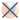实例

<input> 元素最小值与最大值设置:

Enter a date before 1980-01-01:
<input type="date" name="bday" max="1979-12-31">

Enter a date after 2000-01-01:
<input type="date" name="bday" min="2000-01-02">

Quantity (between 1 and 5):
<input type="number" name="quantity" min="1" max="5">

<input> multiple 属性

multiple 属性是一个 boolean 属性.

multiple 属性规定<input> 元素中可选择多个值。实例

Select images: <input type="file" name="img" multiple>

<input> pattern 属性

pattern 属性描述了一个正则表达式用于验证<input> 元素的值。实例

Country code: <input type="text" name="country_code" pattern="[A-Za-z]{3}" title="Three letter country code">

<input> placeholder 属性

placeholder 属性提供一种提示（hint），描述输入域所期待的值。实例

input 字段提示文本t:

<input type="text" name="fname" placeholder="First name">

<input> required 属性

required 属性是一个 boolean 属性.

required 属性规定必须在提交之前填写输入域（不能为空）。实例

Username: <input type="text" name="usrname" required>

<input> step 属性

step 属性为输入域规定合法的数字间隔。实例

<input type="number" name="points" step="3">

HTML5 <input> 标签

<form> 定义一个form表单
<input> 定义一个 input 域

您可能还喜欢：